# Expand and Simplify Double Brackets

In this worksheet, students will expand double brackets and expand where possible.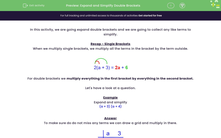Key stage:  KS 3

Curriculum topic:   Algebra

Curriculum subtopic:   Simplify Algebraic Expressions to Maintain Equivalence

Difficulty level:#### Worksheet Overview

In this activity, we are going expand double brackets and we are going to collect any like terms to simplify.

Recap - Single Brackets

When we multiply single brackets, we multiply all the terms in the bracket by the term outside.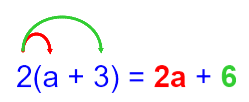For double brackets we multiply everything in the first bracket by everything in the second bracket.

Let's have a look at a question.

Example

Expand and simplify

## (a + 3) (a + 4)

To make sure do do not miss any terms we can draw a grid and multiply in there.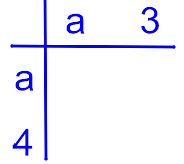Multiply each term together a x a, a x 3, a x 4, 3 x 4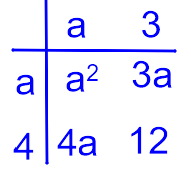There are four terms in the answer as you can see, but the 4a and 3a are like terms and can be added together to leave just three terms in the answer.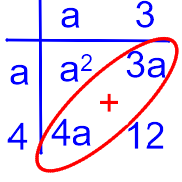Therefore, the answer is a2 + 7a + 12Let's try some questions!

### What is EdPlace?

We're your National Curriculum aligned online education content provider helping each child succeed in English, maths and science from year 1 to GCSE. With an EdPlace account you’ll be able to track and measure progress, helping each child achieve their best. We build confidence and attainment by personalising each child’s learning at a level that suits them.

Get started